# Optimizer Quick Start

This tutorial shows how to:

• Set up a basic NLP optimization model using Decision, Objective, and Constraint Nodes
• Define the central Optimization node using the DefineOptimization function
• Obtain solution output and status
• Specify domain types (i.e., integer, continuous, etc.) and bounds for decisions
• Combine parametric analysis with optimization
• Change initial guesses for non-convex solution spaces

## Structured Optimization

Structured Optimization simplifies the process of formulating a model for optimization. The function, DefineOptimization, uses a similar structure for Linear Programs (LP), Quadratic Programs (QP) and Nonlinear Programs (NLP). It analyzes the model and automatically selects the optimization engine most appropriate for your model. (You can still override this process if you want.)

This section includes simple NLP examples to demonstrate the roles of Decision variables, Constraints, Objectives, and Decision attributes in the Structured Optimization framework. The same basic structure applies equally to LP and QP formulations.

## The Optimum Can Example

The Optimum Can example determines the dimensions of a cylindrical object having a minimum surface area for a given volume. Admittedly, this is not a very interesting optimization problem. In fact, the solution can be derived on paper using basic differential calculus. However, the simplicity of the example allows us to focus on the workflow and object relationships using the new Structured Optimization framework in Analytica.

In this example, we will decide on the Radius and Height of a cylindrical vessel. We represent each of these as a Decision variable in the influence diagram. The values we define for these nodes will be used as initial guesses for optimization types that require an initial guess (NLP or non-convex QP). Otherwise, the definitions of these inputs are not important. We use 1cm as an initial guess for both the radius and height.

Decision Radius := 1
Decision Height := 1

Constants have no special interpretation in optimization definitions. They can be used as usual for values that stay constant in the model. In this example, we will use a Constant for the required volume which does not vary in the model.

Constant Required_Volume := 1000

General variables are used for intermediate values as well as for the central DefineOptimization function described below. We also use a variable to define Volume of the cylinder.

Variable Volume := pi*Radius^2*Height

Constraints contain equality or inequality conditions that restrict the range of optimized results. In this example, we use a constraint object to enforce the minimum volume requirement on our can.

Constraint Volume_Constraint := (Volume >= Required_Volume)

Most optimizations have an objective value to maximize or minimize. (Some problems are only concerned with feasible solutions that meet constraints.) In this example we are minimizing the surface area of our can. We define surface area using an Objective variable. The can has round disks at the top and base with surface area (πR2) and a tubular side with surface area (2πRH).

Objective Surface_area := 2*(pi*Radius^2) + (2*pi*Radius*Height)

### The DefineOptimization() Function

The DefineOptimization() function is the key component of all Structured Optimization models. It brings all other components together, specifying the optimization to be performed. This function is typically placed in a Variable object in the center of our influence diagram. This function includes many optional parameters, but we use only the core parameters in this example:

• Decision:
Identifier for the decision node (or a list of identifiers separated by commas if there are multiple decisions). Specify All to include all decision nodes in the model or All in module to include all desired decisions within a designated module.
• Constraint:
Identifier for the constraint node (or a list of identifiers separated by commas if there are multiple constraints). Specify All to include all constraint nodes in the model or All in module to include all desired constraints within a designated module. You can also specify inequality or equality expressions directly, or omit the parameter entirely in an unconstrained optimization problem.
• Maximize/Minimize:
Use the words “Maximize” or “Minimize” depending on the type of problem. Follow this with an expression or with the identifier for the relevant objective node.

We specify our DefineOptimization node as:

 Variable Opt :=
DefineOptimization(
Constraints: Volume_Constraint,
Minimize: Surface_area)


### Viewing the Optimization Object

The DefineOptimization function evaluates to a special object that contains detailed information about the optimization. The object appears as a blue hyperlink that shows the type of optimization problem you have constructed. In this case we see it is NLP . You can double-click the optimization object to open a new window revealing internal details from the optimization engine. Clicking reference objects allows you to drill down to finer levels of detail. This information is also available by using the OptInfo function.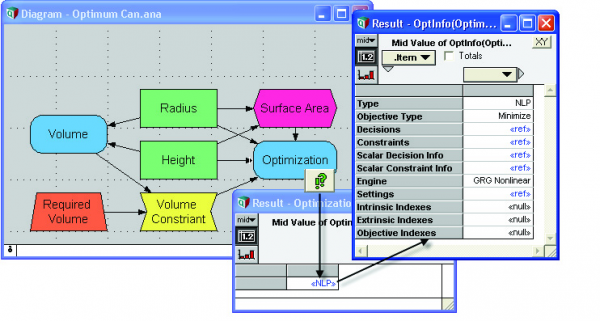In this case, we have allowed Analytica to automatically determine the type of problem. Alternatively, you can specify the problem type along with the desired engine and other settings by adding optional parameters to DefineOptimization. See Optimizer Functions for more details about the Type and Engine parameters of DefineOptimization.

### Obtaining the Solution

Now that we have specified an optimization, how do we compute and view the result? You may be tempted to re-evaluate the Radius and Height decision variables to see if their values have changed. But this is not how optimization works in Analytica. Input values always retain their original definitions. (In this case, we simply used 1 as a dummy value for Radius and Height.) To obtain the solution, you need to create an output node defined with the OptSolution function. This function usually uses two parameters:

OptSolution(Opt, Decision)

• Opt: Identifier for the node containing DefineOptimization
• Decision: Identifier for the counterpart Decision input node

Decision Opt_Radius := OptSolution(Opt, Radius)
Decision Opt_Height := OptSolution(Opt, Height)

The Decision parameter is optional. If it is omitted, the solution will include all decisions along a local index named .DecisionVector ..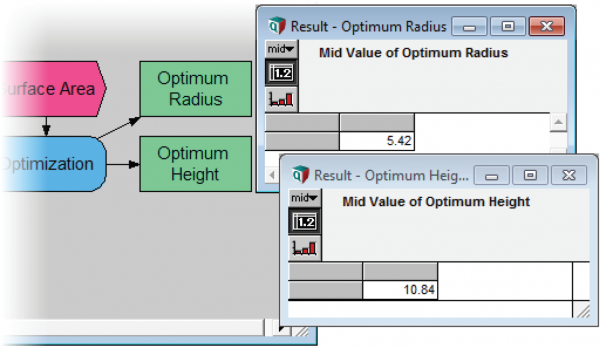### Obtaining the Optimized Objective Value

To conveniently evaluate the optimized objective value (the surface area of the solution can) you can use the OptObjective function. The only parameter is the identifier for the Define Optimization node.

Objective Opt_Surface := OptObjective(Opt)

### Viewing Optimization Status

To check the status of an optimization, use the OptStatusText function. Enter the identifier for the node containing DefineOptimization.

Variable Status := OptStatusText(Opt)

This will reveal a text string describing the status of the optimization result. Status messages differ according to problem characteristics and the engine being used. In general these messages indicate whether or not a feasible solution has been found and if so, whether or not the optimizer was able to converge to a bounded solution. In this example status is: “Optimal solution has been found.”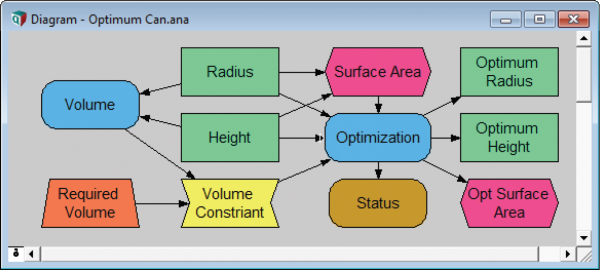### Copying Optimized Results to Definitions

In some cases, you may wish to copy the optimized decision values into the definition of the original decisions. With this, the result for variables downstream of the decisions will reflect their optimal values as well.

You can configure your model to copy optimized results into the original decisions by adding two buttons to your model. The first button solves for the optimal solution and copy the optimal values. The second button restores the original (non-optimized) definition. Functions provided in the Structured Optimization Tool.ana library take care of the details.

To configure these buttons:

1. With the diagram in focus, select Add Library from the File menu.
2. Select Structured Optimization Tools.ana and click Open.
3. Select Embed, then click OK.
4. Drag a button from the tool bar, title it "Set to Optimal."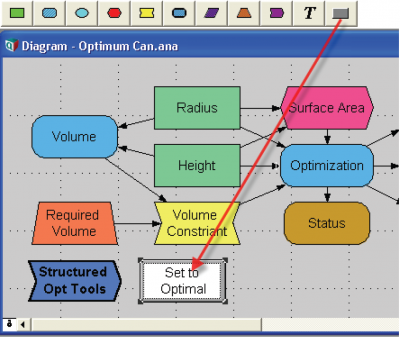1. Clickto edit the button’s Script attribute to Use_opt_decisions(opt).
1. Drag a second button to the diagram, name it "Restore Defintions" and set its Script attribute to Restore_Decision_Defs(opt).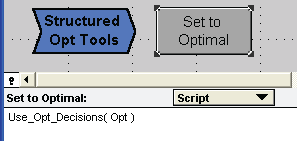Now we’re ready to try them out.

1. Clickto enter browse mode. Click the Set to Optimal button.
2. Open the object window for Radius:### Changing Variable Types (Domain)

Click either Radius or Height to open the Object window for the node. You will notice a pull-down menu for Domain. This attribute specifies the variable type. It is always visible for decision nodes if you are using the Optimizer edition.

Suppose the factory requires Radius and Height to be integer values in centimeters for tooling purposes, or because they don’t like decimals. Change the Domains of Radius and Height to Integer and re-evaluate the solution: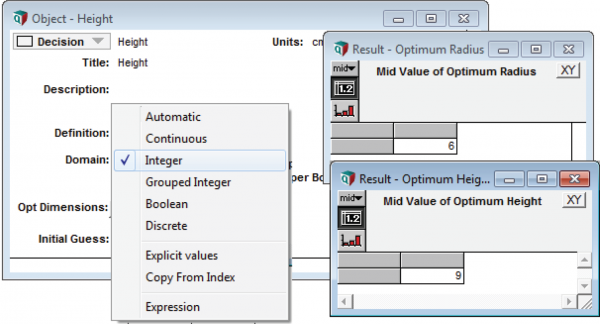The new solution finds the integer values that come closest to meeting the optimization criteria.

See Optimizer Attributes for descriptions of all available domains.

### Setting Bounds on Decision Values

Suppose the cans must not exceed a 5cm radius in order to meet National Association for the Advancement of People with Small Hands (NAAPSH) guidelines. One way to set this limit would be to add another constraint. But since this restriction applies directly to one of the decision variables, it is easier to simply set an upper bound on the variable directly.

Double-click the Radius variable and enter 5 as the upper bound. The updated solution will describe a thinner can that is 5cm in Radius and 13cm in Height.

Bounds and Domains

Some Domain types are not compatible with bounds. If one of these domains is selected (i.e. Boolean), bounds attributes will not be visible.

Bounds and Feasible Solutions

It is possible to have no feasible solution within the designated bounds. For example, if you restrict Radius to 5cm while restricting Height to 10cm, it will be impossible to produce a can that meets the minimum volume constraint. The OptStatusText() function indicates whether or not a feasible solution has been found.

### Using Parametric Analysis with Optimization

Before adding optimization to existing models, it is often useful to perform a parametric analysis to see how variations in decision inputs affect the objective value. If you have done this, your Decision and Objective variables will include parametric indexes. To demonstrate this in the Optimum Can example, we can define the Radius to be a sequence of values that vary parametrically. We then re-define Height such that the volume of the cylinder remains constant as radius varies:

Variable Radius := Sequence(4.5, 6.5, 0.1)
Variable Height := Required_volume/(pi*Radius^2)

Now you can evaluate the objective Surface_Area to see how it is affected by Radius.An optimization requires a scalar-valued objective. An array-valued objective usually implies an array of optimizations, each optimizing an individual element of the objective array. But parametric indexes are an exception to this rule! If the Objective is an array over parametric indexes, the indexes are ignored by the optimization. So even though we have an array valued Objective in this example, there is still only one optimization run.Parametric analysis is a good way to gain insight into your model. The Structured Optimization framework is designed so that it will not be confused by this

### The Initial Guess Attribute

LP and convex QP problems do not rely on initial guesses and always yield a solution that is globally optimal. But in NLP and non-convex QP problems it is not always possible to guarantee that a solution found by the optimizer is a global optimum. It might be merely a “local” optimum within the solution space. Optimization methods for these problems use an initial guess from which to start the search for a solution. The particular solution the optimizer returns may depend on the starting point.

Normally, Analytica uses the defined value of the Decision variables as the initial guess. In the Optimum Can example, we initially defined Radius and Height as 1. If a decision variable is defined using a parametric index, Analytica uses the first element of the parametric array as the initial guess.

You can change the initial guess without re-defining the decision variable using the Initial Guess attribute in the Decision node. We can demonstrate this using the Polynomial NLP.ana example where the objective is a non-convex curve with local maxima.

The Initial Guess attribute is hidden by default. To make it visible in Decision nodes:

• Select Attributes... from the Object menu.
• Check the Initial Guess box.

The attribute will now be visible in the Object windows of all Decision variables.

The polynomial curve in this model is designed to have several critical points.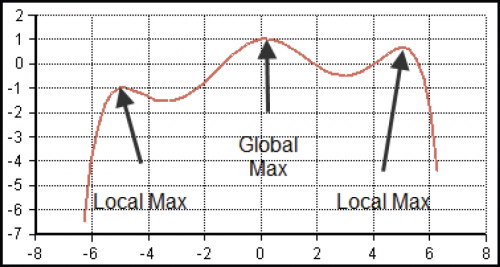Decision X := 0 (or any value at all)
Initial Guess of X := [-4, 2, 0, 2, 4]
Objective Polynomial :=
1 + X/6-X^2/2 + X^4/24 - X^6/720 + X^8/40320 - X^10/3628800
Variable Opt := DefineOptimization(
Decision: X,
Maximize: Polynomial)
Variable X_solution := OptSolution(Opt, X)
Objective Max_Objective := OptObjective(Opt)


The array of initial guesses will cause Analytica to abstract over the index and perform multiple optimizations.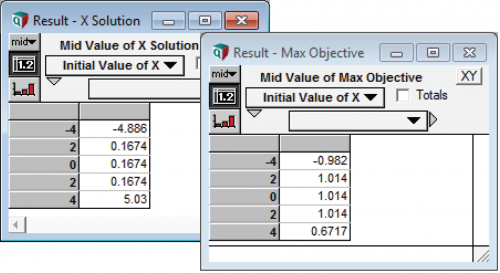We see that the result depends on the initial guess for this non-convex NLP.

If the array of guesses were entered as a definition for the decision variable instead of as an initial guess attribute, Analytica would interpret it as a parametric index and apply only one initial guess. (See subsection above.) Therefore, it is necessary to use the Initial Guess parameter if you want to perform multiple optimizations using an array of guesses.

Note
Finding multiple different local extrema in this fashion can be a useful way to locate multiple solutions of interest. One often needs to combine unmodeled factors with insight and results obtained from a model, so these other solutions may turn out to be more interesting for reasons that you have not modeled.
Note
When your interest is in finding just the global optima, there are additional methods for dealing with the problem of local optima. The Multistart and topological search options can be utilized with gradient-based methods (see Coping with Local Optima). The “Evolutionary” and “OptQuest” engines use population-based search methods that are more robust to local optima.

## Summary of Optimum Can example

This Optimum Can example demonstrates how to formulate and analyze an optimization problem. It includes input Decision variables, a Constraint, an Objective, intermediate Variables and the central DefineOptimization function.

The DefineOptimization function recognizes the non-linear characteristics of the Optimum Can model and classifies it as an NLP. The function evaluates as a special object containing details about the optimization.

The Domain attributes in Decisions allow setup of variable type and bounds.

Structured Optimization is compatible with a decision variable defined as a parametrically varying sequence.

If the Initial Guess attribute is kept hidden or left blank, Analytica will use the defined value of the decision variable as an initial guess. Users can override this value or enter an array of initial guesses by using the Initial Guess attribute in Decision nodes. This attribute is hidden by default but can be made visible when necessary.